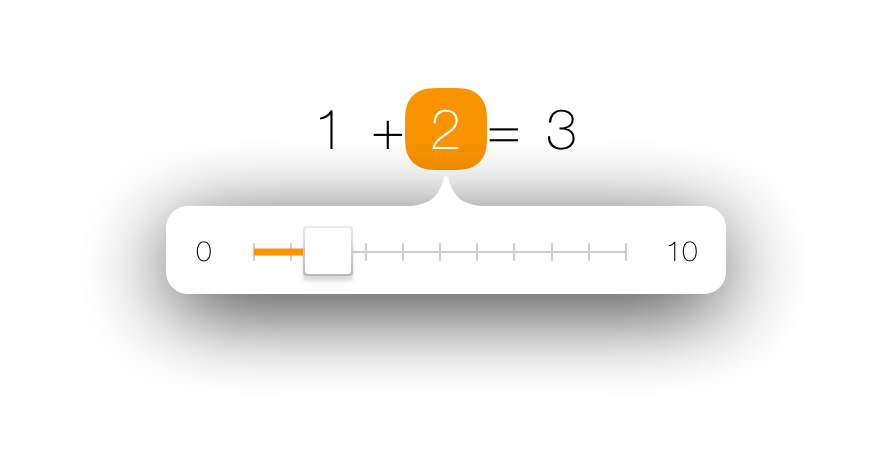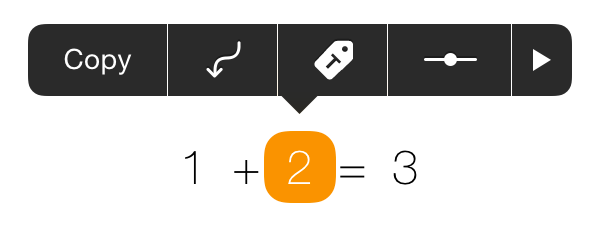# Support

#### Why do results differ from a traditional calculator?

A traditional pocket calculator performs operations in the order they are entered:
(1 + 2) * 3 = 9

Tydlig performs operations in the same order as you would manually, e.g. multiplication and divison before addition and subtraction (PEMDAS):
1 + 2 * 3 = 7

#### How do I graph?Tydlig has a very powerful and flexible graphing function, but it can take some time to get used to. Especially if you are coming from a traditional graphing calculator.

The biggest difference with graphing in Tydlig is the absence of x and y. Instead of writing equations in terms of x and y you write a regular equation with numbers, and connect the numbers you want to "make x and y" to the graph.

There are several ways to do this, the quickest can be seen above. Write an expression like 1 + 2 = 3, long press 1 and tap the graph action. This will insert a graph, connect 1 as x, and 3 as y.

Below are two video examples of how you would graph certain equations in Tydlig.

x + 1 = y

The graph will vary the connected x-number from 0 to 10 in small steps, and draw the resulting y-value at each step.

? is used as a placeholder in the video, it just means some unknown number. You could write any initial number, but sometimes an initial value doesn't make any sense and then ? is a useful placeholder.

The slider is a powerful tool when used in combination with graphs. You can slide any number and see how the graph behaves. In this case the constant 1 is adjusted to show that the graph gets offset up and down.

sin(x) + cos(x + 0.46) = y₁
sin(x * 3.51) * 0.2 = y₂
y₁ + y₂ = y₃

Here we connect multiple x, and also combine equations together. The graph will vary all connected x-numbers from 0 to 10, and draw the value of each connected y at every step. This works regardless of how deep your hierarchy is, or how many x you connect.

The slider is again used to explore how the equations behave when different constants change.

For further information aboute graphing, please see this video review of Tydlig.

#### How do I edit the limits of the graph?

Tap the limit numbers and edit them with the numpad.

#### How are results rounded?

Tydlig rounds results to the nearest number that fits within the specified max decimals. If a result is half way between two possible numbers, it will round away from zero. For example, with two max decimals the result 1.123 would be rounded to 1.12, 1.127 would be rounded to 1.13 and 1.125 which is halfway between 1.12 and 1.13 would be rounded away from zero to 1.13.

Numbers are only rounded for display on screen, behind the scenes the full available precision is always used. So if you link multiple results together you will not lose any precision due to intermediate rounding.

#### How do I save?

Tydlig will automatically save the canvas between launches. There is currently no support for multiple canvases.

It is also possible to export the canvas as a high quality PDF. This works especially well together with apps like Dropbox and Evernote that can store the file for later reference.

### Tips and Tricks

#### Clear buttonTo quickly clear all content, swipe left on the erase button to reveal a red button. Tap the red button to delete everything.

#### Drag whole lineTo easily drag a whole line without selecting it, drag the equals sign (=) and all other numbers on the line will follow along.

#### Discrete sliderTap on the slider to make it snap to whole numbers, tap again to toggle back. You can also tap on the limit numbers and edit them with the numpad.